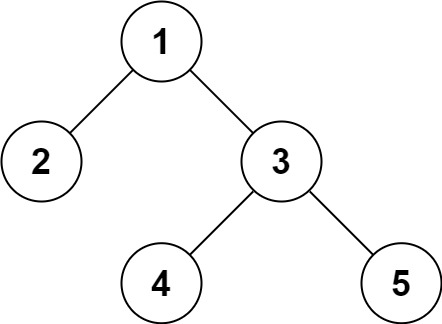# LeetCode: 297. Serialize and Deserialize Binary Tree

## 题目¶

Serialization is the process of converting a data structure or object into a sequence of bits so that it can be stored in a file or memory buffer, or transmitted across a network connection link to be reconstructed later in the same or another computer environment.

Design an algorithm to serialize and deserialize a binary tree. There is no restriction on how your serialization/deserialization algorithm should work. You just need to ensure that a binary tree can be serialized to a string and this string can be deserialized to the original tree structure.

Clarification: The input/output format is the same as how LeetCode serializes a binary tree . You do not necessarily need to follow this format, so please be creative and come up with different approaches yourself.

Example 1:```Input: root = [1,2,3,null,null,4,5]
Output: [1,2,3,null,null,4,5]
```

Example 2:

```Input: root = []
Output: []
```

Example 3:

```Input: root = 
Output: 
```

Example 4:

```Input: root = [1,2]
Output: [1,2]
```

Constraints:

• The number of nodes in the tree is in the range [0, 10^4].
• -1000 <= Node.val <= 1000

## 解法¶

• [1,null,2] 将序列化为 1 N 2 N N
• [2,1,3] 将序列化为 2 1 N N 3 N N
• [5,3,6,2,4,null,7] 将序列化为 5 3 2 N N 4 N N 6 N 7 N N

```# Definition for a binary tree node.
# class TreeNode(object):
#     def __init__(self, x):
#         self.val = x
#         self.left = None
#         self.right = None

class Codec:

def serialize(self, root):
"""Encodes a tree to a single string."""
if root is None:
return 'N'

val = '{}'.format(root.val)
left = self.serialize(root.left)
right = self.serialize(root.right)

return '{} {} {}'.format(val, left, right)

def deserialize(self, data):
"""Decodes your encoded data to tree."""
values = data.split()
root = self._build_tree(values)

return root

def _build_tree(self, values):
if not values:
return None

next_value = values.pop(0)
if next_value == 'N':
return None

val = int(next_value)
root = TreeNode(val)
root.left = self._build_tree(values)
root.right = self._build_tree(values)

return root

# Your Codec object will be instantiated and called as such:
# ser = Codec()
# deser = Codec()
# ans = deser.deserialize(ser.serialize(root))
```